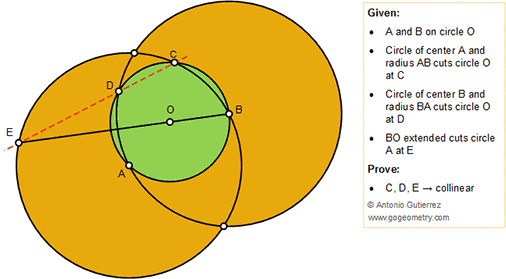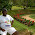## Sunday, December 2, 2018

### Geometry Problem 1404: Three Circles, Center, Intersecting Points, Collinearity, Congruence

Geometry Problem. Post your solution in the comment box below.
Level: Mathematics Education, High School, Honors Geometry, College.

Details: Click on the figure below.#### 4 comments:

1.Let BA extended meet circle A at F

Since BD = AC, ABCD is an isosceles Trapezoid with AB//CD.

So < ACF = < AFC = < DCF = ϴ …… (1) say

Hence < ACD = 2ϴ = < ABD and since ΔOBD ≡ ΔOBA (SSS), < DBO = < ABO = ϴ

Now since < EBF = < ECF = ϴ = DCF from (1)

It follows that C,D,E are collinear

Sumith Peiris
Moratuwa
Sri Lanka

2.Extend DC to F ( F on B)
Tr EAC = Tr BDF (congruent) => Tr EAD = Tr BCF => ang EDA = ang BCF

3.BD = BA = AE = R and AD perpendicular to EB => DBAE rhombus
=> ang CDB + BDA + ADE = arc (CB)/2 + (BA)/2 + (AD + DC)/2 = 180

4.From same point D (DBAE rhombus) we can draw just a paralelel to AB